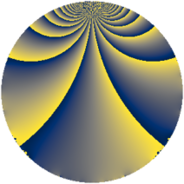# Properties

 Label 1694.2.iLevel $1694$ Weight $2$ Character orbit 1694.i Rep. character $\chi_{1694}(241,\cdot)$ Character field $\Q(\zeta_{6})$ Dimension $144$ Sturm bound $528$

# Related objects

## Defining parameters

 Level: $$N$$ $$=$$ $$1694 = 2 \cdot 7 \cdot 11^{2}$$ Weight: $$k$$ $$=$$ $$2$$ Character orbit: $$[\chi]$$ $$=$$ 1694.i (of order $$6$$ and degree $$2$$) Character conductor: $$\operatorname{cond}(\chi)$$ $$=$$ $$77$$ Character field: $$\Q(\zeta_{6})$$ Sturm bound: $$528$$

## Dimensions

The following table gives the dimensions of various subspaces of $$M_{2}(1694, [\chi])$$.

Total New Old
Modular forms 576 144 432
Cusp forms 480 144 336
Eisenstein series 96 0 96

## Trace form

 $$144q + 72q^{4} + 12q^{5} + 64q^{9} + O(q^{10})$$ $$144q + 72q^{4} + 12q^{5} + 64q^{9} + 8q^{14} + 8q^{15} - 72q^{16} - 16q^{23} + 80q^{25} + 36q^{26} + 12q^{31} + 128q^{36} + 12q^{37} - 12q^{38} - 4q^{42} + 108q^{45} - 36q^{47} - 12q^{49} + 32q^{53} + 4q^{56} + 8q^{58} - 60q^{59} + 4q^{60} - 144q^{64} - 8q^{67} - 68q^{70} - 8q^{71} - 84q^{75} - 12q^{80} - 80q^{81} + 24q^{82} - 28q^{86} - 108q^{89} - 116q^{91} - 32q^{92} - 20q^{93} + O(q^{100})$$

## Decomposition of $$S_{2}^{\mathrm{new}}(1694, [\chi])$$ into newform subspaces

The newforms in this space have not yet been added to the LMFDB.

## Decomposition of $$S_{2}^{\mathrm{old}}(1694, [\chi])$$ into lower level spaces

$$S_{2}^{\mathrm{old}}(1694, [\chi]) \cong$$ $$S_{2}^{\mathrm{new}}(77, [\chi])$$$$^{\oplus 4}$$$$\oplus$$$$S_{2}^{\mathrm{new}}(154, [\chi])$$$$^{\oplus 2}$$$$\oplus$$$$S_{2}^{\mathrm{new}}(847, [\chi])$$$$^{\oplus 2}$$